ControlNet

AI绘画在这几个月火了起来，它能从提供的文字和图片中生成新的绘画，质量很高，而且非常有趣。这个封面就是用AI生成的。但是在使用AI绘画的过程中，搞不懂steps，sampler之类的意思。为了想要更好的使用AI绘画，也想要理解AI绘画中那些参数的含义，所以本着学习新技术的目的，写了这篇文章来学习一下AI绘画。

## AI绘画

masterpiece,best quality,1 girl,loli, small breasts,animal ears, cute, Age:12, Height:145, hair ribbon, cute face,shy, long hair, white hair, dress , illustration, city
Negative prompt: nsfw, lowres, bad anatomy, bad hands, text, error, missing fingers, extra digit, fewer digits, cropped, worst quality, low quality, normal quality, jpeg artifacts, signature, watermark, username, blurry,hands
Steps: 40, Sampler: Euler a, CFG scale: 13, Seed: 353263948, Size: 1216x512, Model hash: 6569e224, Clip skip: 2

## DDPM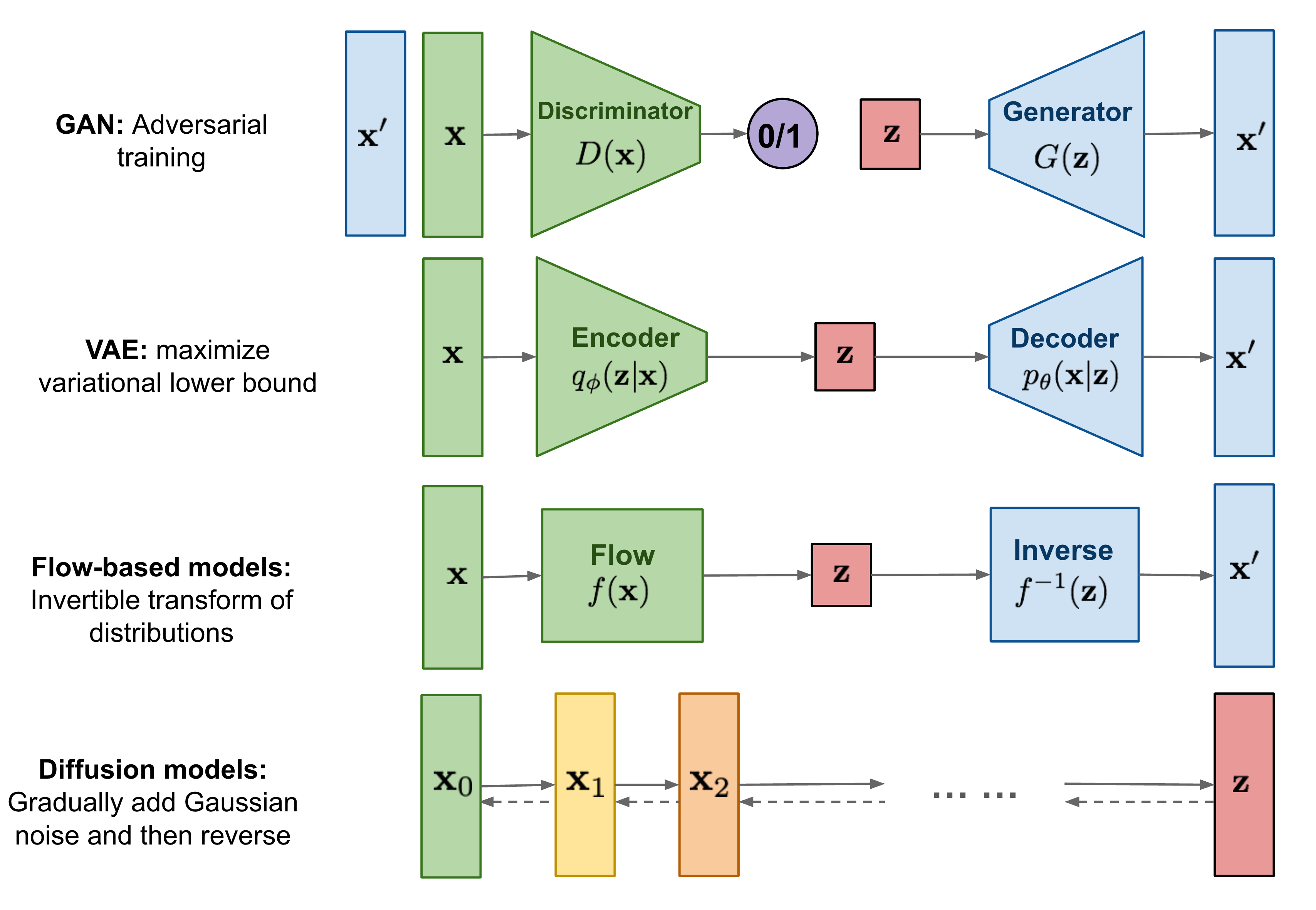Fig. 1. 生成式模型. Adapted from 

DDPM之所以是Diffusion(扩散)，是因为它是通过扩散过程来生成噪声，然后再训练模型去预测这个噪声来去噪，从而达到生成图片的目的。让我们先从扩散的前向过程，也就是生成噪声开始。

### 前向扩散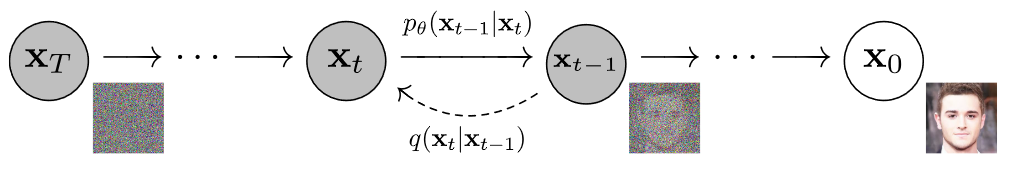Fig. 2. 前向扩散过程. Adapted from 

$$\begin{equation} x_t = \sqrt{\alpha_t}x_{t-1} + \sqrt{1 - \alpha_t}z_t \end{equation}$$

$$\begin{equation} x_t = \sqrt{\prod_{i=1}^t \alpha_i}x_0 + \sqrt{1 - \prod_{i=1}^t \alpha_i}z \end{equation}$$

$$\begin{equation} x_t = \sqrt{\bar{\alpha}_t}x_0 + \sqrt{1 - \bar{\alpha}_t}z \end{equation}$$

### 反向扩散

$$\begin{equation} q(x_{t-1}|x_t,x_0)=\frac{q(x_t|x_{t-1},x_0)q(x_{t-1}|x_0)}{q(x_{t}|x_0)} \end{equation}$$

$$\begin{eqnarray} q(x_{t-1}|x_t,x_0)&\propto& \exp(-\frac{1}{2}((\frac{\alpha_t}{\beta_t}+\frac{1}{1-\bar{\alpha}_{t-1}})x^2_{t-1}-(\frac{2\sqrt{\alpha_t}}{\beta_t}x_t + \frac{2\sqrt{\bar{\alpha_{t-1}}}}{1-\bar{\alpha}_{t-1}}x_0)+C(x_t,x_0))) \\\ &=&\exp(-\frac{(x-\tilde{\mu}_t)^2}{2\tilde{\beta}_tI}) \end{eqnarray}$$

$$\begin{eqnarray} \tilde{\mu}_t &=& \frac{\sqrt{\alpha_t}(1-\bar{\alpha}_{t-1})}{1-\bar{\alpha}_{t}}x_t + \frac{\sqrt{\bar{\alpha}_{t-1}}\beta_t}{1-\bar{\alpha}_t}x_0 \\\ \tilde{\beta}_t &=& \frac{1-\bar{\alpha}_{t-1}}{1-\bar{\alpha}_t}\cdot \beta_t \end{eqnarray}$$

$$\begin{equation} x_0 = \frac{1}{\sqrt{\bar{\alpha}_t}}(x_t - \sqrt{1-\bar{\alpha}_t}\tilde{z}_{t}) \end{equation}$$

$$\begin{equation} \tilde{\mu}_t = \frac{1}{\sqrt{\alpha_t}}(x_t - \frac{1-\alpha_t}{\sqrt{1-\bar{\alpha_t}}}\tilde{z}_{t}) \end{equation}$$

### 训练过程

$$L = ||z_t - \tilde{z}_t||_2$$

### 模型架构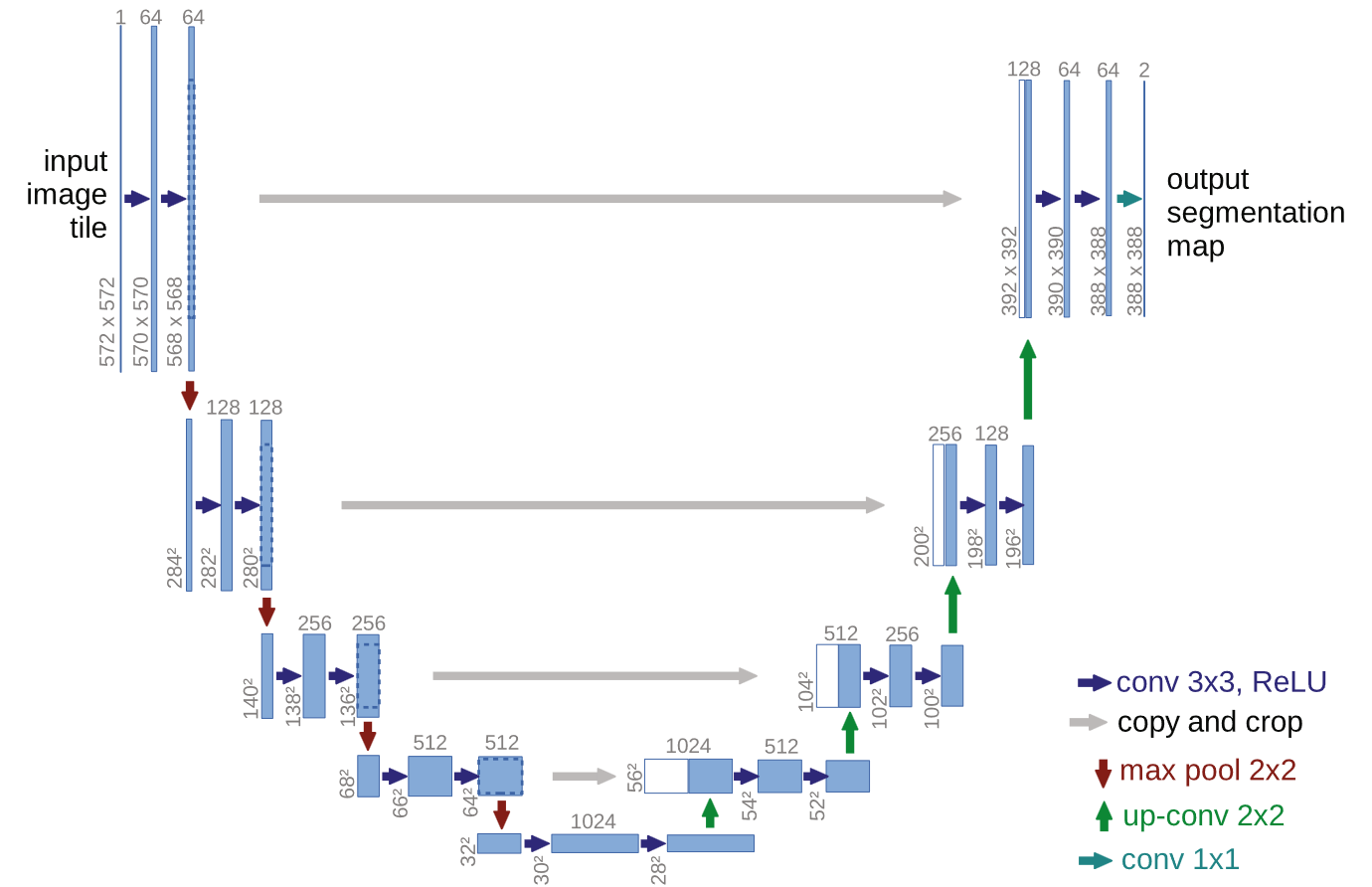Fig. 3. U-Net. Adapted from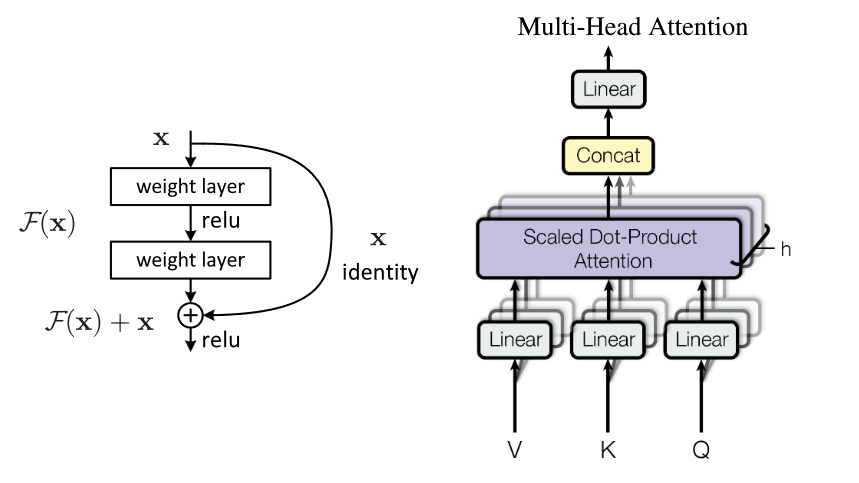### 简单测试结果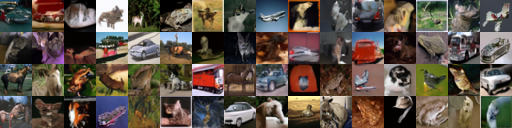Fig. 5. DDPM的简单生成结果

## CLIP

CLIP是由OpenAI提出的多模态预训练算法，它的主要思想是通过一个超大的图像-文本数据集，来训练一个图像Encoder和一个文本Encoder。如果是相关的文本和图片，编码后的特征向量应该是相似的，如果是不相关的文本和图片，编码后的特征向量应该是不相似的。这个数据集有超过4亿个图片和文本的pair，完全是大力出奇迹。

### 模型架构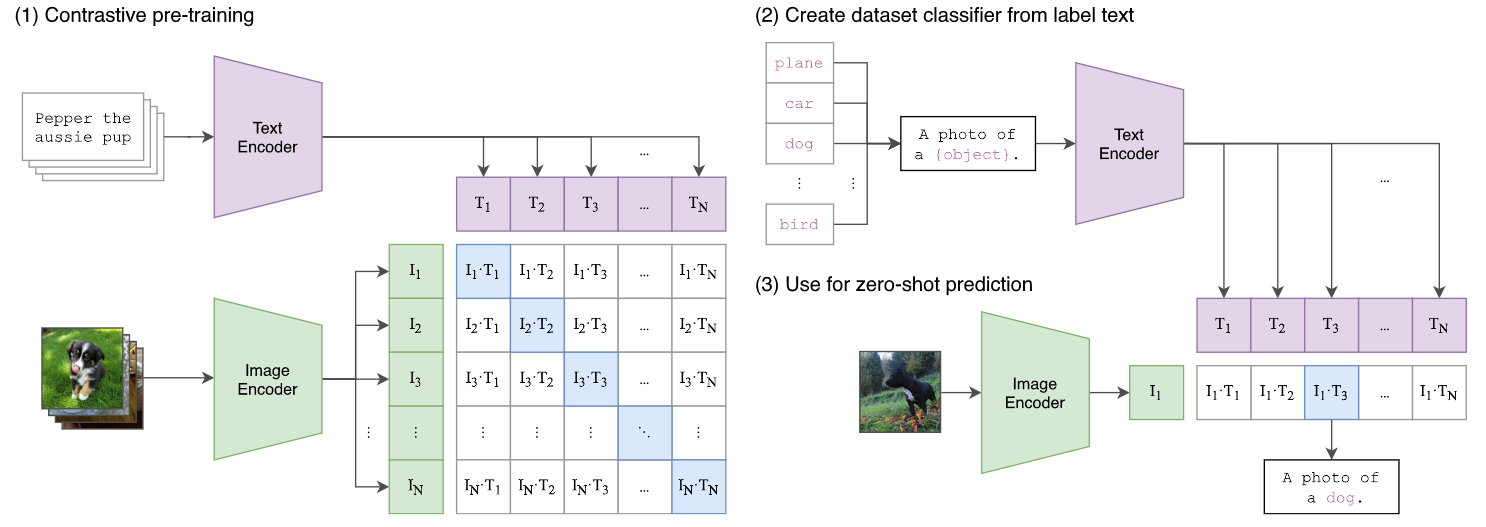Fig. 6. CLIP的模型架构. Adapted from 

### 用于下游任务

CLIP的主要用途是将预训练好的模型用于下游任务。比如说作为zero-shot图像分类任务，如图6(2,3)所示。这个任务很好的展示了这个模型的一大优势，即通过超大的文本图像数据集建立了较为充分的知识，使得模型在没有针对性的训练的情况下，也能够很好的完成下游任务。

## 参考文献

•  Linaqruf, “Linaqruf/anything-v3.0 · Hugging Face,” huggingface.co, 2022. [Online]. Available: https://huggingface.co/Linaqruf/anything-v3.0
•  J. Ho, A. Jain, and P. Abbeel, "Denoising Diffusion Probabilistic Models," in Advances in Neural Information Processing Systems, 2020, vol. 33, pp. 6840–6851. [Online]. Available: https://proceedings.neurips.cc/paper/2020/hash/4c5bcfec8584af0d967f1ab10179ca4b-Abstract.html
•  A. Radford et al., “Learning Transferable Visual Models From Natural Language Supervision,” in Proceedings of the 38th International Conference on Machine Learning, Jul. 2021, pp. 8748–8763. [Online]. Available: https://proceedings.mlr.press/v139/radford21a.html
•  L. Weng, “What are Diffusion Models?,” lilianweng.github.io, Jul. 11, 2021. [Online]. Available: https://lilianweng.github.io/posts/2021-07-11-diffusion-models/
•  O. Ronneberger, P. Fischer, and T. Brox, “U-Net: Convolutional Networks for Biomedical Image Segmentation,” in Medical Image Computing and Computer-Assisted Intervention – MICCAI 2015, Cham, 2015, pp. 234–241. doi: 10.1007/978-3-319-24574-4_28.
•  K. He, X. Zhang, S. Ren, and J. Sun, “Identity Mappings in Deep Residual Networks,” in Computer Vision – ECCV 2016, Cham, 2016, pp. 630–645. doi: 10.1007/978-3-319-46493-0_38.
•  A. Vaswani et al., “Attention is all you need,” in Proceedings of the 31st International Conference on Neural Information Processing Systems, Red Hook, NY, USA, Dec. 2017, pp. 6000–6010.
•  A. Krizhevsky, “Learning Multiple Layers of Features from Tiny Images,” Master’s thesis, University of Tront, 2009.
•  OpenAI, “CLIP,” GitHub, 2022. [Online]. Available: https://github.com/openai/CLIP
•  R. Rombach, A. Blattmann, D. Lorenz, P. Esser, and B. Ommer, “High-Resolution Image Synthesis With Latent Diffusion Models,” in Proceedings of the IEEE/CVF Conference on Computer Vision and Pattern Recognition, 2022, pp. 10684–10695. [Online]. Available: https://openaccess.thecvf.com/content/CVPR2022/html/Rombach_High-Resolution_Image_Synthesis_With_Latent_Diffusion_Models_CVPR_2022_paper.html
•  C. Lu, Y. Zhou, F. Bao, J. Chen, C. Li, and J. Zhu, “DPM-Solver: A Fast ODE Solver for Diffusion Probabilistic Model Sampling in Around 10 Steps,” in Advances in Neural Information Processing Systems, Oct. 2022. [Online]. Available: https://openreview.net/forum?id=2uAaGwlP_V
•  J. Song, C. Meng, and S. Ermon, “Denoising Diffusion Implicit Models,” in International Conference on Learning Representations, Feb. 2022. [Online]. Available: https://openreview.net/forum?id=St1giarCHLP